## Saturday, November 17, 2012

### Was Apple’s Earnings Announcement Really Important?

Many public companies go through the quarterly ritual of announcing their earnings results to the investment community through press releases, conference calls, website slide shows, etc.
There is a lot of disagreement about whether this activity is relevant. On one side are folks who say that without these announcements the company’s share price and valuation will suffer in the investor market place. On the other side are those that believe investors who drive market prices pay attention to long-term cash flow, not quarterly earnings.
Often, share price changes following these announcements are used by management as a gauge as to how the market has responded to the news. We find folks walking around with exaggerated swagger because the stock is up 2% since the conference call, or holed up behind closed doors because it is down 2%.
However, these changes need to be taken with a grain of salt, and in the context of the market in general. Perhaps our stock has gone up 2%, but that can be interpreted quite differently if we also know that the market was up 10% that day, or down 5%.
Fortunately for us, simple statistical tools can tell us something about the relevance of earnings announcements (and other things) to the marketplace.
A Brief Trip Back to Algebra Class
Back in algebra, we learned that there were two equations for a line on a graph, the “point-slope” form and the “slope-intercept” form (see here for more details if you are interested).
A line with the slope-intercept form is depicted by the following equation:
y = mx + bFigure A
In this equation, b is called the intercept because when x is 0, the first term of the equation (m times x) is also 0, and thus b is the only number remaining. It is therefore the value of y when the line intersects the y axis.
The m term is called the slope, because for any value of x, the line will move up or down along the graph incrementally according to that number. The greater the value of m, the more steeply the line will move along the graph.
Figure A shows 2 lines, one with the values m=0.5 and b=3 (the orange line) and the other m=1.5 and b=1 (the brown line). Notice that these lines intersect the y axis at the values of b in the equation, and the lines increase by the value of m for each increase of 1 in the value of x.
In terms of mathematical functions, the slope-intercept form has two variables, the x and the y. Since the value of y depends on what value x holds (remember the line intersects the axis when x is 0, and increases by the amount of change in x), y is known as the dependent variable and x is the independent variable.
AverageFigure B
An average is a measure of the “central tendency” in a set of data. It is obtained by adding all the elements of the data set together and then dividing by the number of items in the data set. The equation for an average is shown in Figure B.
Let’s say we have two sets of data: Set A and Set B. Using the R statistical program, Figure C shows the data set, the two elements of the average formula (sum of items and number of items) and the resulting calculation itself.Figure C
We use two data sets here to illustrate the dangers of averaging. As noted two paragraphs above, an average is supposed to provide an indication of “central tendency” in the data set. We use it often in place of a real figure, or we use its result to anticipate, project, or forecast what future numbers might be - “What is the average temperature in such and such?” “How many sales do we usually have in that region?”
In Figure D we plot the frequency of occurrence of values in each data set and use the dashed lines to represent the resulting average. For Set A, we can see clearly the “central tendency” component – the dashed brown line is in the middle of the “hump of data”.
However, for Set B there are two “humps”, one down near 1 and 2 and the other in the upper teens. The average for Set B is nowhere near any of its data elements! Which implies that the 9.9 average does not at all accurately represent the underlying data!Figure D
So while an average may communicate a realistic assessment of the data, it also may not, and actually serve to mask other dynamics or obscure important information.
The Linear Regression
Linear Regression is a statistical technique that assesses the relationship between two variables. Figure E shows the linear regression equations, with the one at the top of the list the final “product” of the analysis.Figure E
The form of that equation should look suspiciously like the slope-intercept form of a line equation, because that is exactly what it is. Given a scatter plot of points, with one variable on the x-axis and the other on the y-axis, a regression will essentially add a line to the plot (we’ll see this a bit later).
The other thing to note is that the build-up of the regression equations begins with averages (the X bar and Y bar equations), so the cautions we mentioned in the last section apply to this technique.
When I first encountered regression, I thought it was some mystical, rarified technique used by Nobel prize winning scientists. Once I learned more, it became clear that regression is just a fancy way of averaging.
Since I always find equations with subscripts, bars, and Greek letters a bit confusing when first encountered, it helps me to figure out how they work by using real numbers. Figure F shows all of the equations above at work in Excel (I used an example from the Statistical Methods textbook by Snedecor and Cochran). I added arrows to show the flow from one calculation to the next.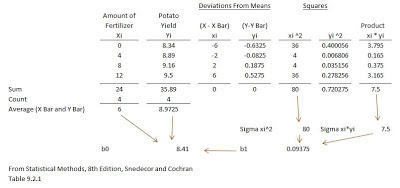Figure F
The final item of note is that the regression equation yields a predicted value for Y, which is the reason for the “hat” symbol on top of the letter in Figure E (oh those mathematicians!). Given actual data, the difference between the actual Y value and the Y hat value is known as the residual.
Using the data in Figure F, the 2nd X value is 4, so the regression equation is:
b0 + b1*X = 8.41 + 0.09375 * 4 = 8.785
This predicted value (the “Y hat” value) is compared to the actual 2nd Y value of 8.89, and the difference between the two is 0.105. (8.89 – 8.785), so this is the residual for this data point.
The Apple Analysis
In order to assess Apple’s earnings announcement impact, we will use the “Market Model” of security prices. The Market Model assumes that for a given change in a stock index, our individual company stock will rise or fall with it to some extent. Thus, the market index is the independent variable, and our individual stock return is the dependent variable.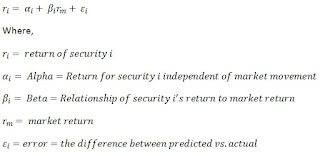Figure G
The Market Model equation for our regression is shown in Figure G. This is nothing more than the equation of a line in slope-intercept form. The only “tweak” we have made to this equation is that instead of using the predicted value of Y (i.e. the “Y hat” value) on the other side of the equals sign we use the actual value of Y. Since we saw in the last section that there is a difference between the predicted and actual values of Y, we account for this difference in the Market Model by use of the ϵ (the “error term”).
Because this model is in slope-intercept form, we can use Regression Analysis to explore the significance of Apple’s earnings announcements.
On July 24, Apple made their earnings announcements for the 2nd Quarter. They did this after the market closed for the day, so the first chance for investors to act on the information was on July 25.
In order to perform our analysis, we divide time into two sections: pre-event and event. In order to avoid “contaminating” the factors we will derive using the regression, we will add a buffer of 5-days between the earnings announcement and the data we use in our regression.Figure H
So in this case since the announcement was made on July 24, we begin excluding data for the regression from July 17th and later.

Figure H shows the regression results of Apple’s daily returns against the NASDAQ (which we use in this example as the stand-in for the “Market Index”) from May 3rd to July 16th.
As we discussed in the prior section, we need to be careful when it comes to averages since the underlying data might not be representative of the averages calculated. One way to assess this visually for regression analysis is to plot the underlying data and then superimpose the regression line. Figure I shows the results of this. This does not look extremely abnormal (except for one data point – do you see it?), which allows us to have a little higher confidence in our calculations.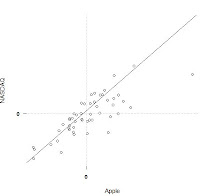Figure I
Now that we have our regression parameters (i.e. the α and β) and have verified that the averaging process has not obscured any major obstacles, we apply these factors to our event time period (July 17th through  August 1st, 5-days after July 25th). From the market return, we calculate using our regression parameters the “predicted” Apple return, and then calculate the residual values (Apple’s actual return vs. the calculated one).
There are several ways we can analyze the results of this. In this post we will focus on the most visual methods, which is simply to examine the market return, apply 2 standard deviations to the predicted Apple return, and then examine whether the residual return is within these parameters or is not. We use 2 standard deviations because that approximates a 95% confidence level in the results.Figure J
The result of this approach is shown in Figure J. The earnings announcement date is represented by the vertical gray line, and we can see that on the day after the residual value was over 2 standard deviations lower than predicted. This would indicate that the earnings announcement was a significant event.
Given that all investors do not immediately respond to announcements, one way to account for this within our regression framework is to look at the cumulative residuals. Figure K shows this approach. On the date of announcement, the residuals were right about where the market returns were, indicating our regression model was almost a perfect predictor by that day.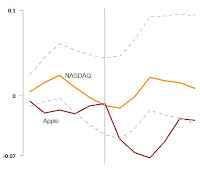Figure K
Again, on the day after the residuals indicated a significant occurrence, but interestingly enough by the 5th day after the cumulative residuals were again within the 2 standard deviation band. So maybe it was not significant after all!
What Can You Do?
There are two things you can do when presented with a regression analysis that will allow you to improve your decision making results. These are:
1. Be a Data Inquisitor – when presented with an average or summary information, inquire into information related to the underlying data structure. Are there histograms available to verify that there is only “one hump”? Are there enough data points to warrant a conclusion? What are the regression related statistics that support any assumed relationships (look at Figure I: R-squared, t-ratios, p-values)?
2. Examine the data in multiple ways – in the Apple analysis we discovered that from one perspective the results were significant, whereas in the other approach they were significant for a few days but by 5 days time they were not. This suggests that an “overlay of judgment” needs to be applied when deciding what conclusions should be drawn. Beware those who are unable or unwilling to show you different looks at the results.
Key Takeaways
Regression can be valuable analytical tool to use in a number of settings within your organization. However, it is not always the appropriate approach, due to issues with the underlying data or results which are not clearly definitive. For these reasons, business leaders need to dig into these areas prior to making decisions.
Questions
What are your experiences where regression analysis let to inaccurate conclusions and/or decisions?
Add to the discussion with your thoughts, comments, questions and feedback! Please share Treasury Café with others. Thank you!

1.2.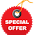3.